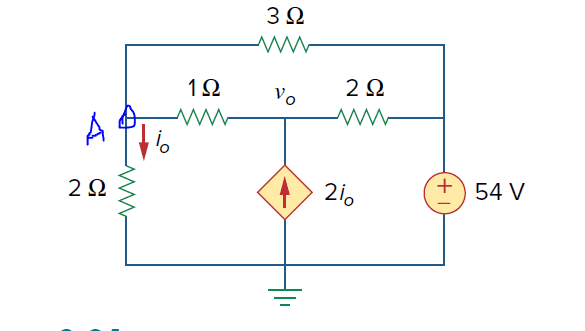# Determine Io and Vo in the circuit

• Engineering
icesalmon
Homework Statement:
Determine io and vo
Relevant Equations:
Ohms law: V=I*R
I am calling the voltage at the node the 2ohm resistor VA
at Node A: ( VA - 54 ) / 3 + ( VA - Vo ) / 1 + (VA - 0) / 2 = 0
at Node Vo: (Vo - VA) / 1 - 2*io = (Vo - 54) / 2
io = VA/2
at Node A: Vo - VA)/1 - 2*(VA/2) = (Vo - 54)/2
Vo = 4*VA - 54
Node A: (VA - 54)/3 + VA - (4*VA - 54) + VA/2 = 0
VA = 16.6V
Vo = 12.4V
io = 8.3A
The solution does not use Node Voltage, instead using a super mesh on the bottom two loops and solving that way which is fine. But it produces a value of VA = 114V and io = 36A.#### Attachments

Mentor
Homework Statement:: Determine io and vo
Relevant Equations:: Ohms law: V=I*R

at Node Vo: (Vo - VA) / 1 - 2*io = (Vo - 54) / 2
This looks like a sign error in the RHS, and why did you use a different form of writing the sum of all currents out of a node is zero? It's best to stick with that form for each equation instead of trying to flip some of the currents, IMO.

•DaveE and icesalmon
icesalmon
This looks like a sign error in the RHS, and why did you use a different form of writing the sum of all currents out of a node is zero? It's best to stick with that form for each equation instead of trying to flip some of the currents, IMO.
Got it, good catch! Thanks! I'm not sure why I do that, my brain just flips between the two as I write the equations out. I wrote it as the sum of the currents being 0 and it worked out great, good tip!

•berkeman
Mentor
Glad that it helped. Did it resolve the discrepancy?

I've found that I need to stay single-minded (and simple-minded) in problems like this. If I try to get creative, it usually comes back to bite me in the end.•DaveE
icesalmon
Glad that it helped. Did it resolve the discrepancy?

I've found that I need to stay single-minded (and simple-minded) in problems like this. If I try to get creative, it usually comes back to bite me in the end.yeah, it yielded the answer from the solution!

•berkeman